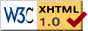Parameter estimation for marked point processes

This study is supervised by Xavier Descombes and Josiane Zerubia, both from the Ariana group, and is partially supported by the French Space Agency (CNES).

Marked point processes are useful for image processing problems such as object extraction from remote sensing images. The advantage of these processes is that they allow the inclusion of strong geometrical constraints on the objects to be detected.

In our applications, a marked point process is defined by a density function with respect to the Poisson measure. Within the framework of Gibbs point process, this density is expressed as a combination of several energy terms: firstly, a data energy term, which controls the localization of the objects with respect to the data; secondly, prior information about the objects is given by internal energy terms corresponding to geometrical constraints on the objects. The weights associated with each internal energy are the so-called `hyperparameters'. These `hyperparameters' used to be calibrated by hand. As their values depend on the kind of images studied, the calibration step is often long. In order to develop fully unsupervised detection procedures, an estimation of these hyperparameters has to be performed.

First of all, an estimation strategy is studied in the case of complete data, for which the configuration, i.e. the set of marked points corresponding to the objects, is known. In this case, several estimators such as maximum likelihood or pseudo-likelihood estimators may be derived. However, since the normalizing constant of the process density is not tractable, maximum likelihood estimators cannot be derived directly. The likelihood is, rather, computed using an importance sampling method. This implies the simulation of importance weights using MCMC methods. MCMCML estimators are then numerically obtained by maximizing the resulting estimated likelihood. Another estimation method is based on the pseudolikelihood, which is a combination of valid likelihoods associated with conditional events. Pseudolikelihood estimators are then obtained by maximizing the corresponding pseudolikelihood. The interest of this last procedure is that one avoids the simulation step, since the normalizing constant does not appear in the pseudolikelihood. Of course, these estimators are sub-optimal, and their performance has to be compared to MCMCML estimators.

Finally, in the more general framework of missing data, i.e. when the configuration is unknown, there is in general no tractable closed form expression for the density associated with the observations. To tackle this problem, estimation methods such as the Expectation-Maximization (EM) can be used. This work is in progress.

 send me an e-mail Last update : 02/05/2008